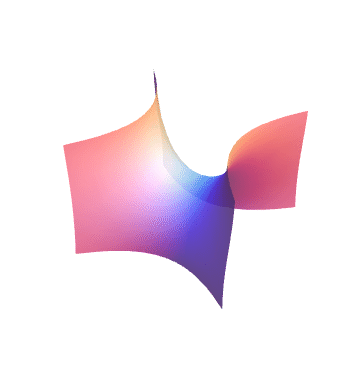Minimal Surfaces in $\mathbb{R}^3$

Weierstrass Representation

Let $M$ be a Riemann surface and $(g,\eta)$ a pair of meromorphic function and holomorphic 1-form on $M$　such that $\big(1+|g|^2\big)^2\eta\overline{\eta}$ gives a Riemannian metric on $M$. Then $f(p)=\mathrm{Re}\int_{p_0}^p\big(1-g^2,\,i(1+g^2),\,2g\big)\eta$ gives a conformal minimal immersion (possibly multi-valued on $M$) into $\mathbb{R}^3$, where $p_0$ is a fixed point on $M$.

Acknowledgements

This site is inspired by Minimal Surface Museum and Minimal Surface Repository maintained by Matthias Weber. I thank him, and I also thank Peter Connor, Shimpei Kobayashi, Wayne Rossman, and Seong-Deog Yang for valuable comments about drawing minimal surfaces.